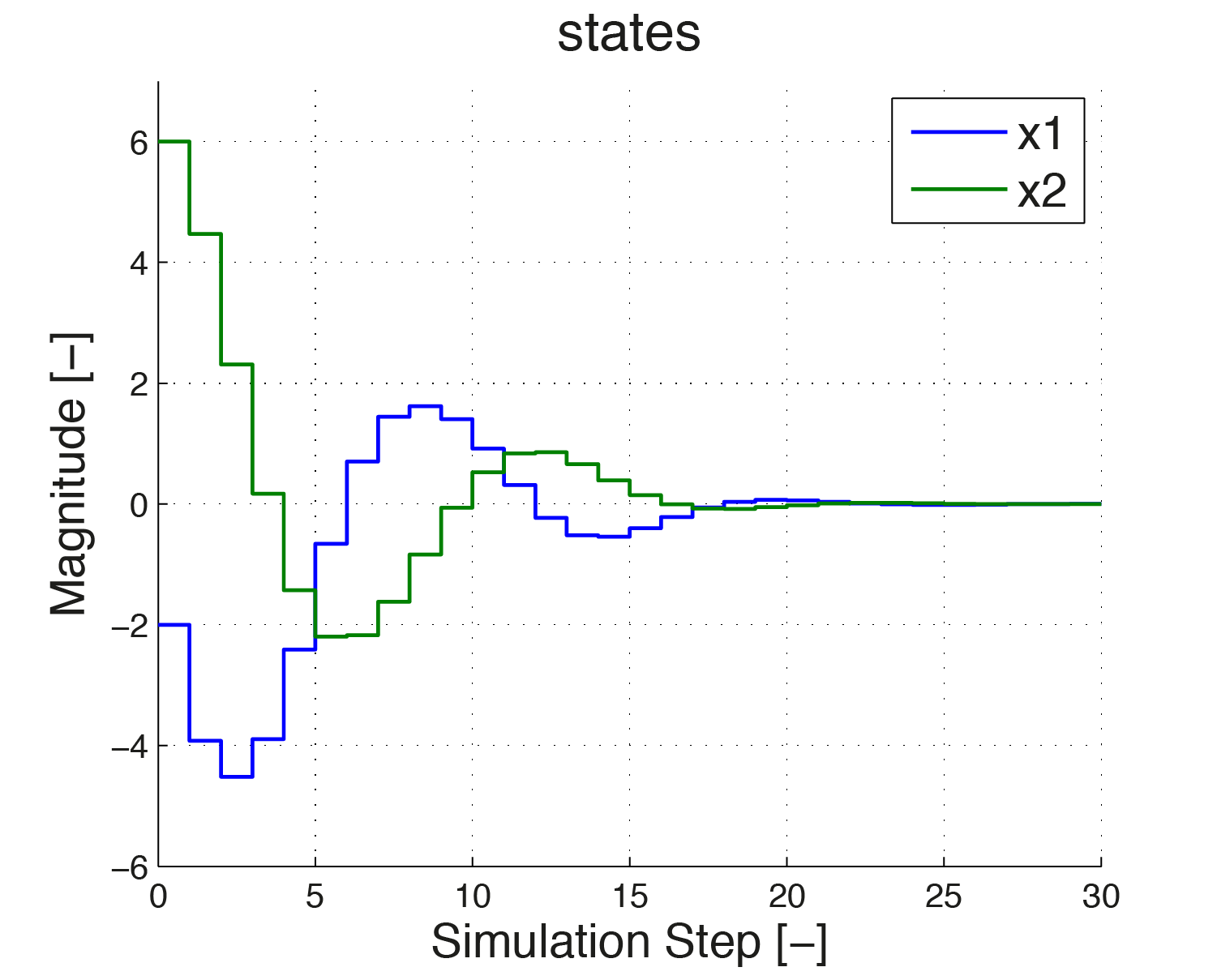# 8.1.5. HOW TO: Implement Rate Constraints¶

## 8.1.5.1. Problem formulation¶

In this example it is illustrated how slew rate constraints on a system’s actuators can be incorporated in the controller design. As a real world example one could think of an airplane, where the elevator cannot be switched instantaneously from one position to another, i. e. has a limited slew rate. Here the concept of constraints on the slew rate is shown on the following system:

\begin{align*} x_{k+1}= \begin{pmatrix} 0.7115 & -0.4345 \\ 0.4345 & 0.8853 \end{pmatrix} x_k + \begin{pmatrix} 0.2173 \\ 0.0573 \end{pmatrix}u_k \Leftrightarrow x_{k+1}=Ax_k +Bu_k \end{align*}

To have a bound on the slew rate, $$u_k − u_{k−1}$$ has to lie in some range, i. e.

$\Delta u_{min} \leq u_k−u_{k−1} \leq \Delta u_{max}.$

One option to set the constraints on the slew rate is to augment the state as follows:

$\begin{split}\hat{x}_{k}= \begin{pmatrix} x_k \\ u_{k-1} \end{pmatrix} \quad \Leftrightarrow \quad \hat{x}_{k+1}= \begin{pmatrix} A & B \\ 0 & I \end{pmatrix}\hat{x}_k + \begin{pmatrix} B \\ I \end{pmatrix}\hat{u}_k \quad \Leftrightarrow \quad \hat{x}_{k+1}= \hat{A} \hat{x}_k + \hat{B}\hat{u}_k\end{split}$

where $$\hat{u}$$ is defined as $$u_k − u_{k−1}$$. To implement the problem using FORCES Pro, the multistage problem has to be defined as stated here. The optimization variable is $$z_i = [ \hat{u}_i \quad \hat{x}_{i+1}]^T$$.

\begin{align*} \hat{x}_{k+1}=& \hat{A} \hat{x}_k + \hat{B}\hat{u}_k \\ \Delta u_{min} &\leq \hat{u} \leq \Delta u_{max} \\ u_{min} \leq& u \leq \Delta u_{max} \\ &\Big\Downarrow \\ \text{minimize}& \quad \frac{1}{2}\sum_{i=1}^{N} z_i^T H_i z_i \\ \text{subject to}& \quad D_1 z_1 = c_1 \\ & \quad C_{i-1}z_{i-1} + D_{i}z_i = c_i \\ & \quad z_{min} \leq z_i \leq z_{max} \end{align*}

The details on how the first equality and the interstage equality look like and how the constraints are implemented can be seen in the MATLAB® code below.

## 8.1.5.2. Implementation¶

%% FORCES multistage form
% assume variable ordering zi = [uhat_i, xhat_{i+1}] for i=1...N-1

stages = MultistageProblem(N); % get stages struct of length N

for i = 1:N

% dimension
stages(i).dims.n = 4; % number of stage variables
stages(i).dims.r = 3; % number of equality constraints
stages(i).dims.l = 2; % number of lower bounds: minimal slew rate and minimal input
stages(i).dims.u = 2; % number of upper bounds: maximal slew rate and maximal input

% cost
if( i == N )
stages(i).cost.H = blkdiag(R_sr, [P, zeros(2,1); zeros(1,2), 0]); % terminal cost (Hessian)
else
stages(i).cost.H = blkdiag(R_sr, [Q, zeros(2,1); zeros(1,2), R]);
end
stages(i).cost.f = zeros(3,1); % linear cost terms

% lower bounds
stages(i).ineq.b.lbidx = [1,4]; % indices of lower bounds
stages(i).ineq.b.lb = [dumin; umin]; % lower bounds

% upper bounds
stages(i).ineq.b.ubidx = [1,4]; % indices of upper bounds
stages(i).ineq.b.ub = [dumax; umax]; % upper bounds

% equality constraints
if( i < N )
stages(i).eq.C = [zeros(3,1), [ A, B; zeros(1, 2), 1]];
end
if( i>1 )
stages(i).eq.c = zeros(3,1);
end
stages(i).eq.D = [[B;1], -eye(3)];

end

% RHS of initial equality constraint is a parameter
parameter(1) = newParam('minusAhat_times_xhat0',1,'eq.c');

% Define outputs of the solver
output(1) = newOutput('uhat',1,1);

% Solver settings
codeoptions = getOptions('RateConstraints_Controller');

% Generate code
generateCode(stages,parameter,codeoptions,output);


You can download the Matlab code of this example to try it out for yourself by clicking here.

## 8.1.5.3. Simulation Results¶

For simulation the following specifications are assumed: the initial condition $$x_0 \in [-2; 6]$$, the input signal $$u$$ is in the range $$[-0.5, 2]$$ and the constraints on the slew rate is $$\hat{u} \in [-1, 0.5]$$. Figure 8.10, Figure 8.11 and Figure 8.12 show how the controller regulates both states to zero while $$\hat{u}$$ and $$u$$ remain in the required range.Figure 8.10 The states are both regulated to zero. No constraints are imposed on the states.

In Figure 8.11 and Figure 8.12 one sees how the input signal is maximally increased in the beginning with a slew rate of $$0.5$$, until it reaches its upper bound of 2. In the figure on the right the slew rate is depicted. One can see that in the beginning, the slew rate stays at its upper bound 0.5. At simulation step 6 the input signal is maximally reduced. Again this is visible from the slew rate being at its lower bound $$-1$$.